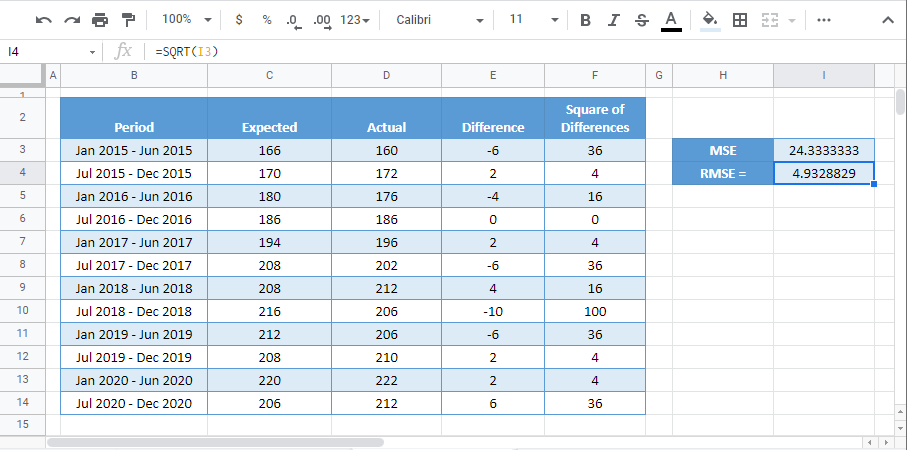# How to Calculate Root Mean Squared Error (RMSE) – Excel and Google Sheets

This tutorial will demonstrate how to calculate Root Mean Squared Error (RMSE) in Excel and Google Sheets.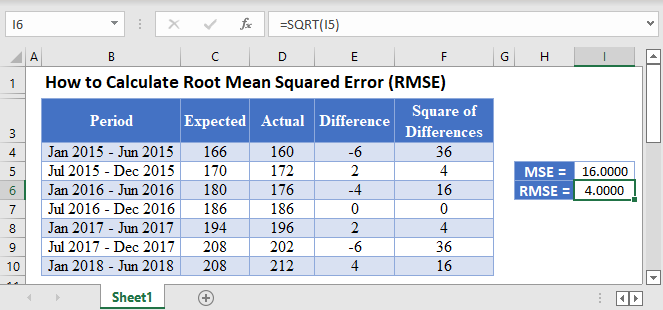The Root Mean Squared Error (RMSE) is an estimate that measures the square root of the average squared difference between the estimated values and the actual values of a dataset.In regression analysis, the RMSE calculates the square root of the average squared differences between the points and the regression line. That is, the square root of the mean of the squares of the residuals.

The RMSE measures the accuracy of forecasting errors produced by different forecasting models for a particular dataset and not between datasets. The RMSE is always positive, and decreases as the error in the forecasts decrease. That is, the closer the predicted values are to the actual values, the smaller the RMSE.

The Root Mean Squared Error (RMSE) is the square root of the Mean Squared Error (MSE). The RMSE of a set of observations is calculated using the formula: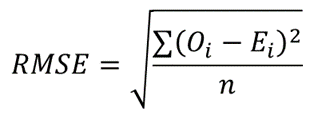where Oi are the observed values;

Ei are the expected values;

∑ is a Greek letter called sigma which represents ‘sum’; and

n is the sample size (the number of observations).

## How to Calculate Root Mean Squared Error in Excel

Background: The table below shows the biannual expected and the actual spending, in thousands of dollars, on residential and non-residential building construction for a certain state in the United States from January 2015 to July 2020.

Using the data in the table, calculate the root mean squared error (RMSE) of the actual data from the forecasted data.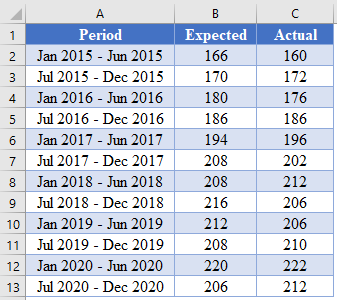### Method 1: SUMSQ Function

First, obtain the difference between the predicted values and the actual values.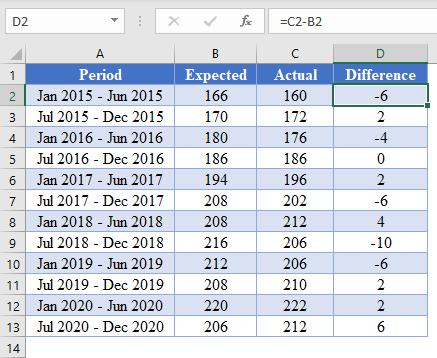Note: Double-Click the bottom right corner of the cell to fill-down the data to the rest of the column.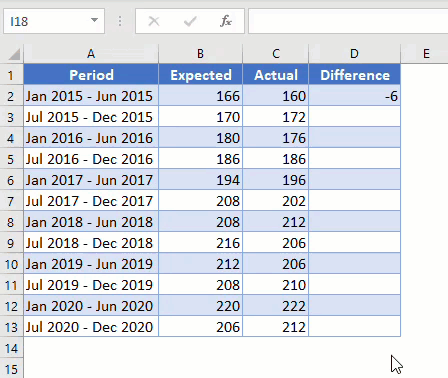Next, calculate the MSE using the SUMSQ Function.

``=SUMSQ(D2:D13)/COUNT(D2:D13)``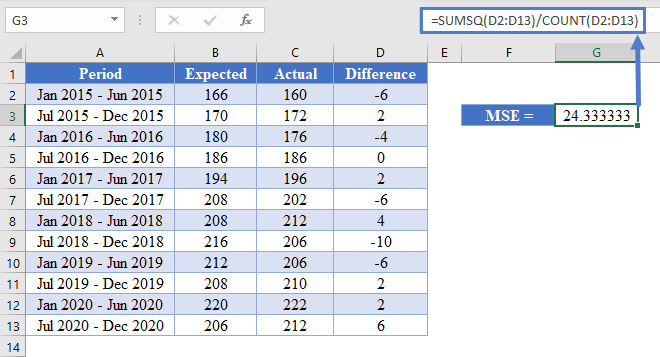Finally, calculate the RMSE by taking the square root of the MSE.

``=SQRT(G3)``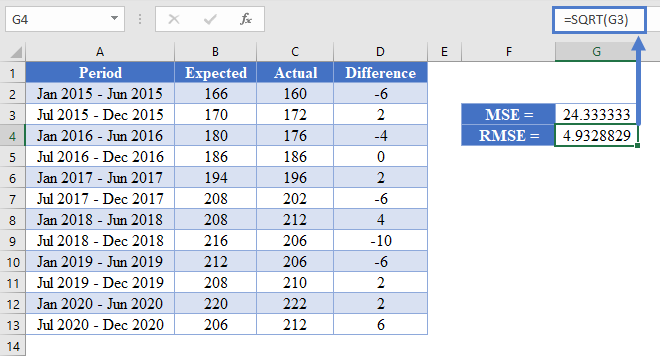### Method 2: AVERAGE Function

Another method you can use to obtain the RMSE of a dataset is using the AVERAGE function. This method is described below.

First, perform the steps described in the method illustrated in the SUMSQ Function method above to obtain the difference between the predicted values and the actual values as shown in the picture below.Next, calculate the square of the differences between the actual and the predicted values.

``=D2^2``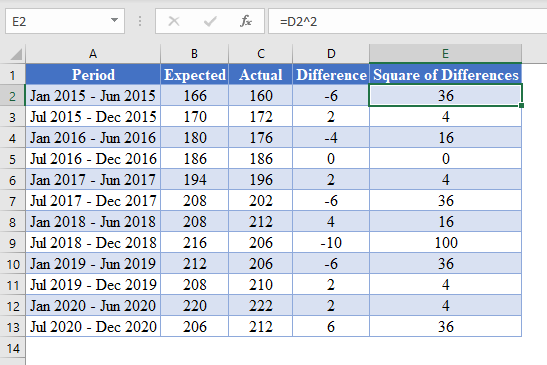Next, calculate the MSE by taking the average of the Square of Differences column.

``=AVERAGE(E2:E13)``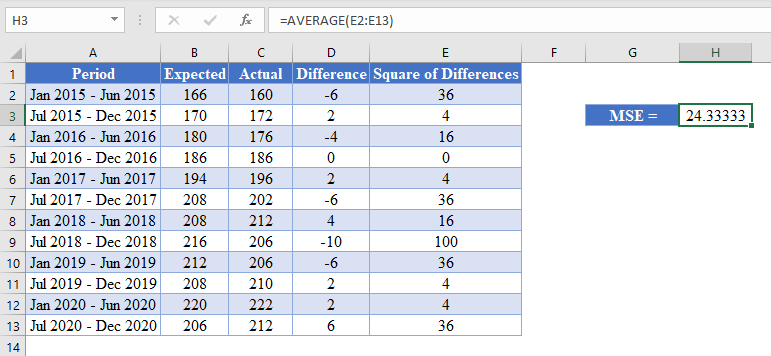Finally, calculate the RMSE by taking the square root of the MSE.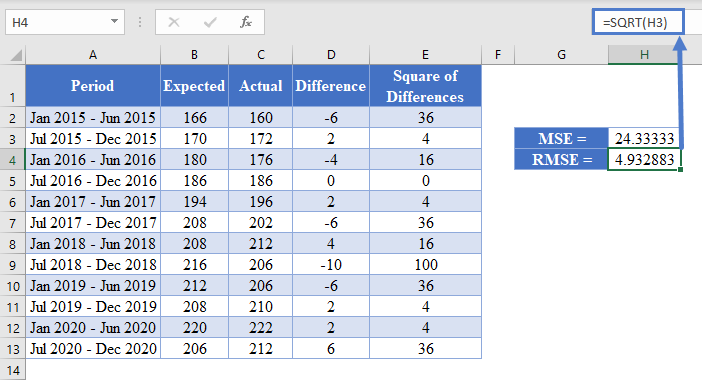### Method 3: The RMSE Formula

Another method you can use to calculate the RMSE of a dataset is using the RMSE formula. This is done by taking sum of the Square of Differences and dividing it by the count (sample size), and then taking the square root of the result as shown in the picture below.

#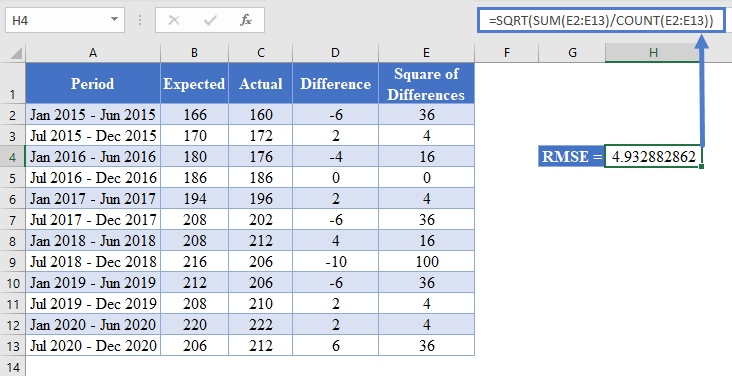## Root Mean Squared Error in Google Sheets

The Root Mean Squared Error (RMSE) can be calculated in Google Sheets in the exact same way as it is calculated in Excel as shown in the pictures below.

First, calculate the MSE as shown in the pictures below.

#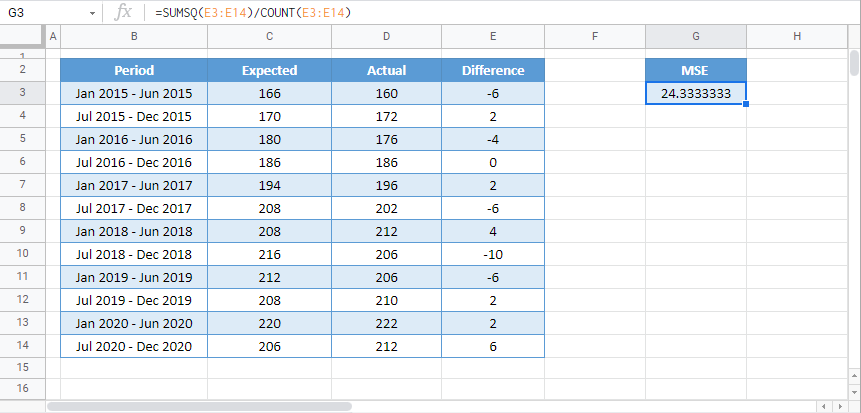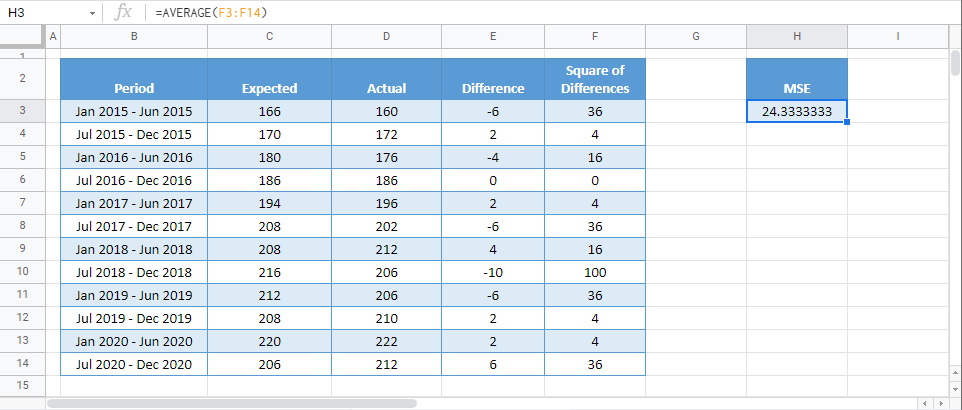Then, calculate the RMSE by taking the square root of the MSE.